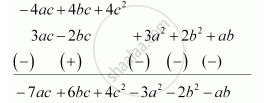Share

# Subtract: 3a (A + B + C) − 2b (A − B + C) from 4c (− A + B + C) - CBSE Class 8 - Mathematics

ConceptMultiplying a Monomial by a Polynomial Multiplying a Monomial by a Trinomial

#### Question

Subtract: 3a (a + b + c) − 2b (a − b + c) from 4c (− a + b + c)

#### Solution

3a (a + b + c) − 2b (a − b + c) = 3a2 +3ab + 3ac − 2ba + 2b2 − 2bc

= 3a2 + 2b+ ab + 3ac − 2bc

4c (− a + b + c) = − 4ac + 4bc + 4c2

Subtracting these expressions, we obtainTherefore, the result is −3a2 −2b2 + 4c2 − ab + 6bc − 7ac.

Is there an error in this question or solution?

#### APPEARS IN

NCERT Solution for Mathematics Textbook for Class 8 (2018 to Current)
Chapter 9: Algebraic Expressions and Identities
Ex. 9.30 | Q: 5.4 | Page no. 146
Solution Subtract: 3a (A + B + C) − 2b (A − B + C) from 4c (− A + B + C) Concept: Multiplying a Monomial by a Polynomial - Multiplying a Monomial by a Trinomial.
S